# What is a Genetic Algorithm?

“It is not the strongest species that survive, nor the most intelligent, but the ones most responsive to change.”

Says Charles Darwin. This is the basis of the genetic algorithm, which is a type of evolutionary algorithm; To adapt to the desired goal. The genetic algorithm is a method used in optimization and search problems based on evolutionary processes in nature. Its basic principle is to eliminate the weakest candidates and keep the strongest ones. It can be applied to problems in almost every field such as applied sciences, engineering, social sciences.

To briefly mention the method, each candidate solution is represented by a chromosome. The genes that make up the chromosome are the values of the candidate solution. The chromosomes in the population interact with each other to generate new generations (cross-over) and try to get closer to the target. Besides, in a process similar to evolution in nature, individuals in the population change (mutation) over time. New individuals formed as a result of these processes continue to be in the population if they are strong; otherwise, they are eliminated for the sake of not reducing the quality of the community. In this way, the genetic algorithm is terminated when the specified target is reached/approached or as a result of a certain number of iterations.

We will proceed with an easy-to-understand example to make the mentioned steps and concepts more clear. Our goal is to create a sequence of random 5 numbers, which makes 100 when added.

Representation of individuals as chromosomes

Individuals in the population are represented by the chromosome concept in biology. The genes that make up the chromosome are the features of individuals. If we adapt our problem to these concepts, a chromosome consisting of the values [1, 23, 67, 12, 52] can be seen in Figure 1.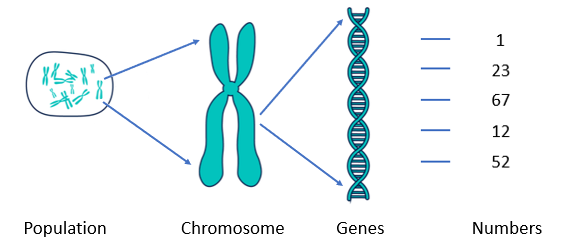Figure 1. Chromosome representation

One of the essential steps in the genetic algorithm is the correct and efficient chromosome representation. This representation directly affects both the efficiency and success of the application.

Population generation

The genes of the chromosomes that will lead to the solution of the problem can be generated randomly at the beginning, or they can be created more intelligently with the logic specific to the problem. With the population created with N number of chromosomes, the model becomes ready for iteration.

Fitness value

How strong/successful the chromosomes (individuals) in the population are must be measured in order to understand how close we are to our goal. In this way, weak chromosomes will be eliminated, the quality of the population will increase and the probability of reaching the target will increase. The most important step in the genetic algorithm problems is to determine what the fitness value is desired to be reached and how it will be calculated. The fitness value of the chromosome in our example can be calculated as | 100–155 | -> 55. The closer the difference is to zero, the stronger that chromosome is for us.

Cross-over

After creating the population, the 2 selected chromosomes interact with each other to produce new chromosomes. This interaction takes place in the form of gene exchange. While our example is our second chromosome [45, 12, 8, 6, 11], these two chromosomes will change some of their genes (numbers) to form new chromosomes.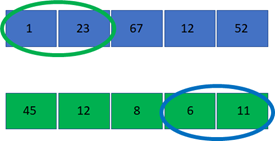Figure 2. Two chromosomes to cross-over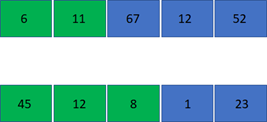Figure 3. New chromosomes formed after cross-over

In the example, the first two genes of the first chromosome and the last two genes of the second chromosome have been replaced (Figure 2). As a result of this process, two new chromosomes with fitness values 48 and 11 are obtained from the two chromosomes with fitness values 55 and 18 (Figure 3). The altered genes can be chosen in different ways or completely at random. Similarly, different methods can be applied to choose which two chromosomes to cross. Just as one approach with a high probability of crossing the strongest chromosomes can be applied, this selection can be completely random.

Mutation

While the chromosomes in the population create new individuals by exchanging genes among themselves (Figure 4), after a certain period of time due to the low gene variety, the population will be attached at the local minimum and stronger chromosomes will not be formed. To solve this problem and increase the diversity in the population, some of the genes of the chromosomes are changed and the chromosomes are mutated. Similar to cross-over, both stronger or weaker individuals may be formed as a result of mutation. Also, the mutation does not have to be made in every iteration. It can also be applied with a certain probability value or in cases where the population is stuck to the local minimum.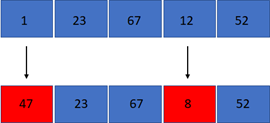Figure 4. Chromosome formed after mutation

Elimination

After the fitness values of new individuals formed as a result of cross-over and mutation are calculated, the weakest candidates (formerly in the population or newly produced) are eliminated to keep the population number constant. In this way, it is ensured that the total success of the population may be increased, and more importantly, it is guaranteed that it will not decrease. If we sum up all the transactions made, the flow is as follows in Figure 5.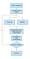Figure 5. Genetic algorithm flow

Example applications

For the problem mentioned above, let’s try to create a chromosome from chromosomes consisting of 5 random numbers, whose numerical values add up to 100. In the following 3 different outputs, respectively; mutation only, cross-over only, cross-over and mutation together were applied. The “Smallest”, “Largest” and “Average” values in the graphs are respectively; shows the most successful, unsuccessful and average fitness values in the population (Figure 6). The third approach produced numbers that reached the desired value in the 48th iteration ([39, 11, 12, 30, 8]). In the second approach, the required value was reached at the 248th iteration ([8, 9, 37, 9, 37]). In the first approach, although the desired value was approached as a result of the 1000th iteration, it was not reached ([22, 1, 10, 14, 55]).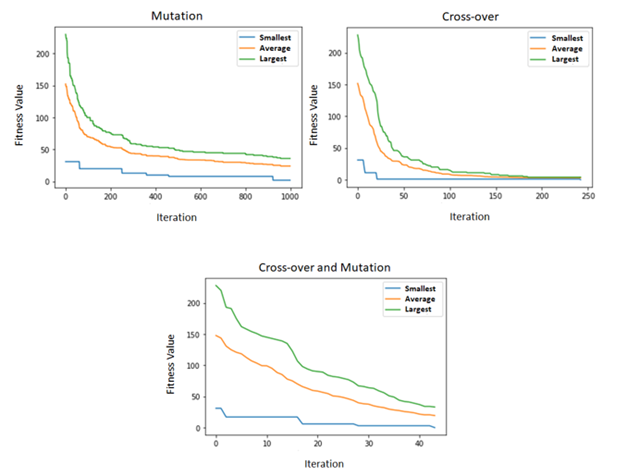Figure 6. Effects of cross-over and mutation

As a result of this experiment, it is seen that using cross-over and mutation together is the most successful method. For the method where the only cross-over is used, the lowest, highest and average fitness values converge as the diversity gradually decreases after gene exchange. Also, using only the cross-over causes the solution to be stuck at a local minimum. The other method with only mutations acts as a random search method and cannot reach the result. In the case where mutation and crossing are used together, the diversity increases thanks to the mutation and the strongest fitness value, which is blue, does not remain stationary as in the second method.

Let’s do a second example to illustrate the application of the genetic algorithm in a different field, although it has no purpose as a practice. This time, our goal is to get a target sentence from random letters. As the fitness value, let’s use the Leveinstein distance, which indicates how many changes need to be made to get from one expression to the other one. The smaller this distance, the closer the two expressions will be. We are trying to converge to the target of the genetic algorithm (“genetic algorithm is actually pretty cool”), which is established with a structure similar to the previous example. Figure 7 shows the population’s strongest chromosome in each iteration and the fitness value of that chromosome. In this way, we achieve the goal by getting better step by step.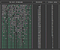Figure 7. Steps to converge to the target sentence

Finally, for another example, let’s try to reach a target image (Figure 8) by using random pixel values.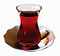Figure 8. Target image to be created

In this problem, we take the difference between the chromosomes and the pixels in the original image as the fitness value. The smaller the difference, the closer we are to the original image. The strongest chromosomes in different iterations and the improvement in the model are shown in the figure below.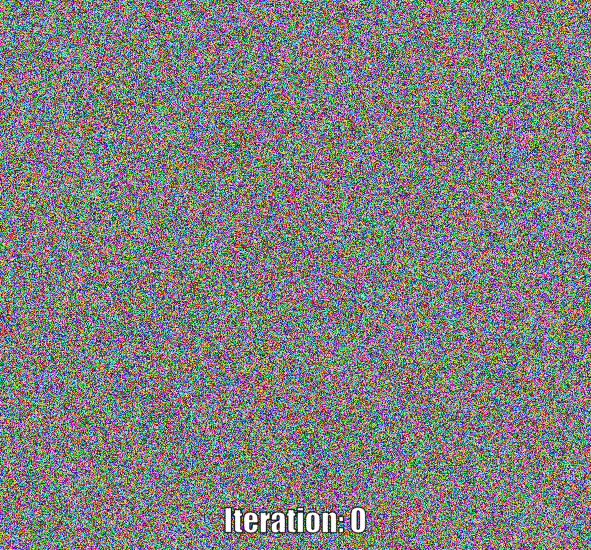Strongest chromosomes in different iterations

The first two applications were implemented not in the most efficient but a straightforward way in order to follow the working logic of genetic algorithms better without using a library. Yet, the code for the last example was developed using the PyGAD to show how to use a library for genetic algorithms. You can access these codes of the examples on GitHub; example1, example2, example3.

Teşekkürler:)